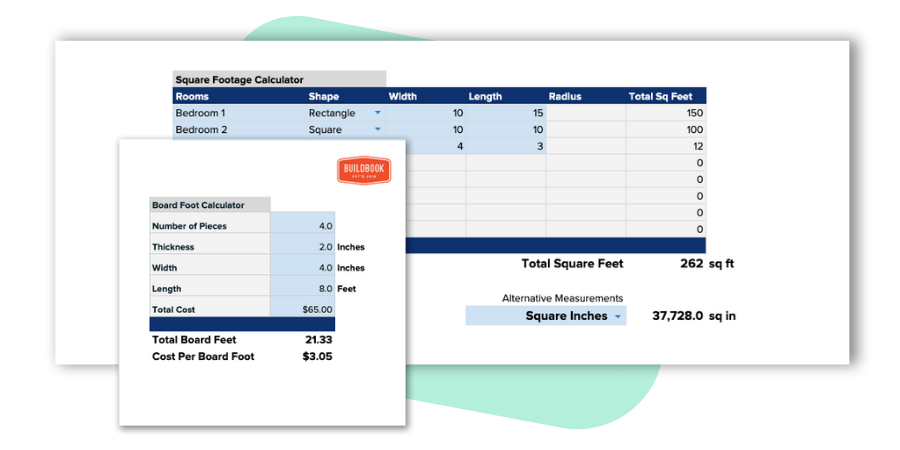# Framing CalculatorQuickly determine the number of studs you need and the total cost to build a wall

This collection of construction calculators is 100% free to download, copy, and use as your own. You're free to modify and/or adjust any calculator to best fit the needs of you or your business.This framing calculator is designed to help residential builders and remodelers easily calculate the total cost and amount of lumber needed to frame a single wall. The calculator factors in on-center spacing between studs, lumber wastage, top and bottom plates, and using multiple studs at wall ends.

## How to calculate the number of studs you need to construct a wall

The two most important factors you need to determine the number of studs (2x4's) you need for a wall are; 1. The width of the wall, and 2. the on-center spacing between your studs, or in other words the distance between the centers of each stud.

With these two numbers, the formula is pretty simple:

Number of studs needed = (Wall Width / On-Center Spacing)+ 1

For example, if your wall is 10' wide and distance between your studs is 16", the formula would be (10/1.33)+1, or (120/16)+1. Since your width is in feet and your spacing is in inches, you'll need to convert one of them into the same measurement. Whichever option you choose, the final result is the same, 8.5 studs are needed.

But, you may not be done yet.

### Top and bottom plates

The formula above accounts for the vertical studs you'll need, but does not include the horizontal studs running along the top and bottom of your wall, or rather your plates.

To determine the number of studs you'll need for your plates, you'll first need to decide if you'll want a single stud plate or more. In other words, the number of studs layered together for each plate.

Assuming we're using a double stud layer for each plate, the formula is as follows:

Plate studs needed: (Wall Width / Stud Length) * 4

Continuing with our example above, and assuming we're using 8 foot studs, the formula would be (10 / 8) * 4, or 1.25 * 4. Based on this, we'll need an additional 5 studs, for a new total of 18.5 studs (8.5 + 5).

### Material waste

Material waste in any construction project is a near certainty. If you don't account for it, you'll likely come up short when it comes to completing the project.

The percentage of material waste can vary greatly in projects, but 15% is a fairly common percentage to factor in if you're unsure.

The formula to account for additional waste is as follows:

Total Studs * (Waste Percentage / 100 + 1)

If we estimate that we'll need to factor for 15% waste on the 11 studs we need, our formula would be 11 * (15 / 100 + 1), or 11 * 1.15 which gives us a total of 12.65 studs.